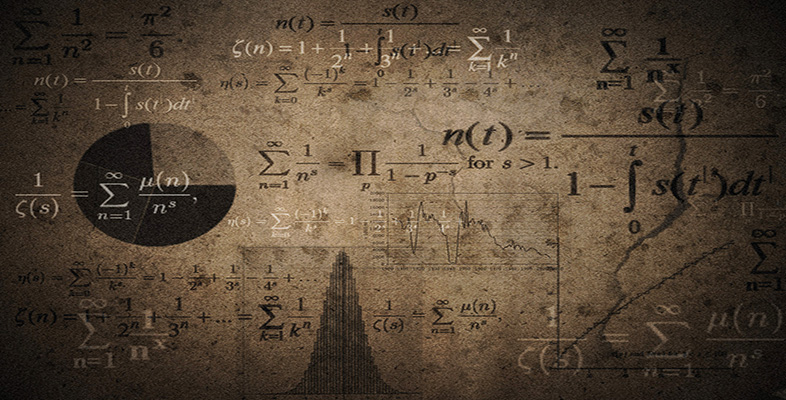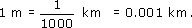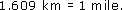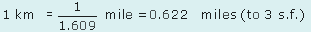Language, notation and formulas

This free course is available to start right now. Review the full course description and key learning outcomes and create an account and enrol if you want a free statement of participation.

Free course

# 3.4 Converting units by using a formula

It is sometimes necessary to convert from one unit of measurement to another. You can use a formula in order to do this.

## Example 11

Use the formula 1 pint = 0.5683 litres (to 4 s.f.) to calculate the number of litres in 8 pints (or 1 gallon). Give your answer to 3 significant figures.

As 1 pint is 0.5683 litre, it follows that 8 pints will be 8 times this:

8 pints = 8 × (0.5683 litre) = 4.55 litres (to 3 s.f.).

Sometimes the conversion formula that is given is in the opposite direction from the one you want. However, it is possible to turn such formulas round. For example, since 1 km = 1000 m, it follows that## Example 12

Turn the formula 1 mile = 1.609 km round to obtain 1 km in miles. Give your answer to 3 significant figures.

The formula can be written asDividing by 1.609 givesMU120_4M6